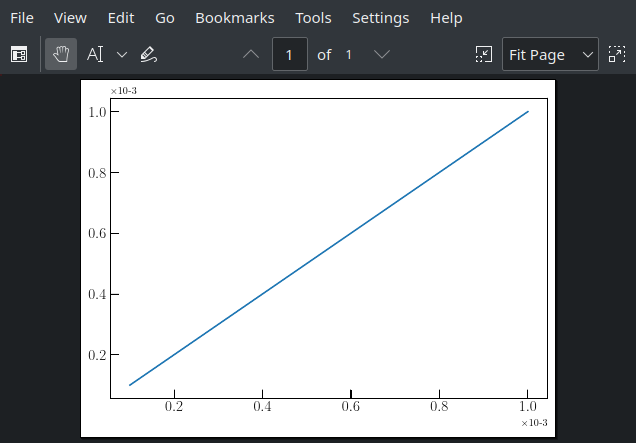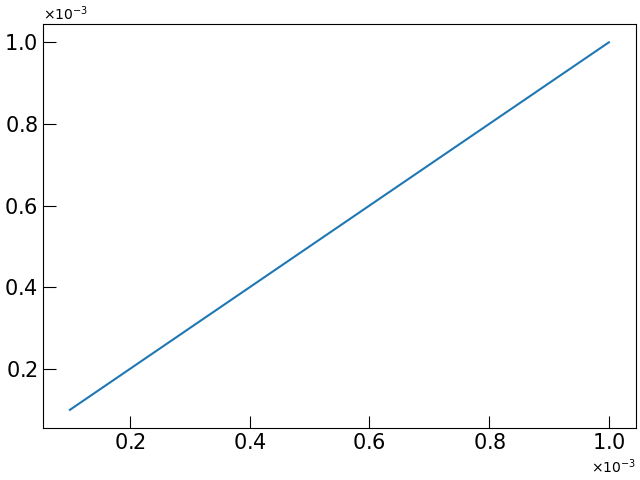# Formatting tick labels with useMathText produces bad PGF plot

When I set AX.ticklabel_format(useMathText=True), and save as a PGF plot, it gets stuck later when compiling with pdflatex, saying:

 ! Missing } inserted
<inserted text>
}
l.277 ...selectfont $$\displaystyle \times{10^{− 1}}{}$$}%
! Extra }, or forgotten $. l.277 ...lectfont $$\displaystyle \times{10^{−1} }{}$$}%  A minimum (not)working example is as follows. Uncomment all the commented lines in order to save a PGF file. #import matplotlib as mpl import matplotlib.pyplot as plt import numpy as np #mpl.use("pgf") #PGF_WITH_RC_FONTS = { # "font.family": "serif", # "font.serif": [], # "font.sans-serif": [], #} #mpl.rcParams.update(PGF_WITH_RC_FONTS) FIG, AXES = plt.subplots(constrained_layout=True) X = np.linspace(0.0001, 0.001, 10) AXES.plot(X, X) AXES.tick_params(direction='in', length=9, labelsize=15) AXES.tick_params(which='minor', direction='in') AXES.ticklabel_format(style='sci', scilimits=(0, 1), useMathText=True) #plt.savefig('matplotlib_useMathText.pgf', bbox_inches='tight') plt.show()  The images produced in the PDF (upon skipping through the errors in pdflatex), compared against what one may see normally:As you can see, the “10^{-3}” is not rendered correctly in the case of the PDF derived from the PGF. The latex code that generates the pdf using pdflatex is as follows: \documentclass{standalone} \usepackage{amsmath,amssymb} \usepackage[T1]{fontenc} \usepackage[utf8]{inputenc} \usepackage{lmodern} \usepackage{pgf} \DeclareUnicodeCharacter{2212}{$-\$}

\begin{document}
\input{matplotlib_useMathText.pgf}
\end{document}


Solution courtesy of Antony Lee (Gitter):

Use \DeclareUnicodeCharacter{2212}{\ensuremath{-}}.